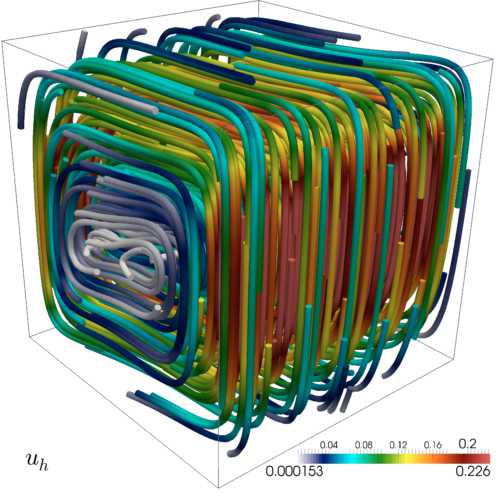﻿﻿ CI²MA - Research | Fundamental Areas | Numerical Analysis of Partial Differential Equations

### Fundamental Areas / Numerical Analysis of Partial Differential Equations

20 October 2014: Numerical simulation of a coupled flow-transport problemThese figures show the computed temperature iso-surfaces (left) and velocity streamlines (right) arising from the numerical simulation of a benchmark test of thermal convection on the cube [0,1]^3. The corresponding numerical method is given by an augmented mixed finite element method for the fluid coupled with a usual primal finite element method for the transport equation. Further details are available in:

 CI²MA, CENTER FOR RESEARCH IN MATHEMATICAL ENGINEERING, UNIVERSIDAD DE CONCEPCIÓN - MAILBOX 160-C, CONCEPCIÓN, CHILE, PHONE: +56-41-2661324